### One more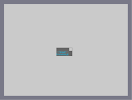Hover over the thumbnail for a full-size version.

Author RavenMac author:ravenmac n-art nonplayable rated 2009-08-26 2009-08-26 4 by 12 people. \$One more#RavenMac#nonplayable#00000000000000000000000000000000000000000000000000000000000000000000000000000000000000000000000000000000000000000000000000000000000000000000000000000000000000000000000000000000000000000000000000000000000000000000000000000000000000000000000000000000000000000000000000000000000000000000000000000000000000000000000000000000000000000000000000000000000000000000000000000000000000000000000000000000000000000000000000000000000000000000000000000000000000000000000000000000000000000000000000000000000000000000000000000000000000000000000000000000000000000000000000000000000000000000000000000000000000000000000000000000000000000000000000000000000000000000000000000000000000000000000000000000000000000000000000000000000000000|1^348,326!1^349,326!1^350,326!1^351,326!1^352,326!1^353,326!1^354,326!1^355,326!1^356,326!1^357,326!1^358,326!1^359,326!1^360,326!1^361,326!1^362,326!1^363,326!1^364,326!1^365,326!1^366,326!1^367,326!1^368,326!1^369,326!1^370,326!1^371,326!1^372,326!1^373,326!1^374,326!1^375,326!1^376,326!1^377,326!1^378,326!1^379,326!1^380,326!1^381,326!1^382,326!1^383,326!1^384,326!1^385,326!1^386,326!1^387,326!1^388,326!1^389,326!1^390,326!1^391,326!1^392,326!1^393,326!1^394,326!1^395,326!1^396,326!1^397,326!1^398,326!1^399,326!1^400,326!1^401,326!1^402,326!1^403,326!1^404,326!1^405,326!1^406,326!1^407,326!1^408,326!1^409,326!1^410,326!1^411,326!1^412,326!1^413,326!1^414,326!1^415,326!1^416,326!1^417,326!1^418,326!1^419,326!1^420,326!1^421,326!1^422,326!1^423,326!1^424,326!1^348,325!1^349,325!1^350,325!1^351,325!1^352,325!1^353,325!1^354,325!1^355,325!1^356,325!1^357,325!1^358,325!1^359,325!1^360,325!1^361,325!1^362,325!1^363,325!1^364,325!1^365,325!1^366,325!1^367,325!1^368,325!1^369,325!1^370,325!1^371,325!1^372,325!1^373,325!1^374,325!1^375,325!1^376,325!1^377,325!1^378,325!1^379,325!1^380,325!1^381,325!1^382,325!1^383,325!1^384,325!1^385,325!1^386,325!1^387,325!1^388,325!1^389,325!1^390,325!1^391,325!1^392,325!1^393,325!1^394,325!1^395,325!1^396,325!1^397,325!1^398,325!1^399,325!1^400,325!1^401,325!1^402,325!1^403,325!1^404,325!1^405,325!1^406,325!1^407,325!1^408,325!1^409,325!8^409,325,1!1^411,325!1^412,325!1^413,325!1^414,325!1^415,325!1^416,325!1^417,325!1^418,325!1^419,325!1^420,325!1^421,325!1^422,325!1^423,325!1^424,325!1^348,324!1^349,324!1^350,324!1^351,324!1^352,324!1^353,324!1^354,324!1^355,324!1^356,324!1^357,324!1^358,324!1^359,324!1^360,324!1^361,324!1^362,324!1^363,324!1^364,324!1^365,324!1^366,324!1^367,324!1^368,324!1^369,324!1^370,324!1^371,324!1^372,324!1^373,324!1^374,324!1^375,324!1^376,324!1^377,324!1^378,324!1^379,324!1^380,324!1^381,324!1^382,324!1^383,324!1^384,324!1^385,324!1^386,324!1^387,324!1^388,324!1^389,324!1^390,324!1^391,324!1^392,324!1^393,324!1^394,324!1^395,324!1^396,324!1^397,324!1^398,324!1^399,324!1^400,324!1^401,324!1^402,324!1^403,324!1^404,324!1^405,324!1^406,324!1^407,324!1^408,324!1^409,324!8^409,324,1!1^411,324!1^412,324!1^413,324!1^414,324!1^415,324!1^416,324!1^417,324!1^418,324!1^419,324!1^420,324!1^421,324!1^422,324!1^423,324!1^424,324!1^348,323!1^349,323!1^350,323!1^351,323!1^352,323!1^353,323!1^354,323!1^355,323!1^356,323!1^357,323!1^358,323!1^359,323!1^360,323!1^361,323!1^362,323!1^363,323!1^364,323!1^365,323!1^366,323!1^367,323!1^368,323!1^369,323!1^370,323!1^371,323!1^372,323!1^373,323!1^374,323!1^375,323!1^376,323!1^377,323!1^378,323!1^379,323!1^380,323!1^381,323!1^382,323!1^383,323!1^384,323!1^385,323!1^386,323!1^387,323!1^388,323!1^389,323!1^390,323!1^391,323!1^392,323!1^393,323!1^394,323!1^395,323!1^396,323!1^397,323!1^398,323!1^399,323!1^400,323!1^401,323!1^402,323!1^403,323!1^404,323!1^405,323!1^406,323!1^407,323!1^408,323!1^409,323!8^409,323,1!1^411,323!1^412,323!1^413,323!1^414,323!1^415,323!1^416,323!1^417,323!1^418,323!1^419,323!1^420,323!1^421,323!1^422,323!1^423,323!1^424,323!1^348,322!1^349,322!1^350,322!1^351,322!1^352,322!1^353,322!1^354,322!1^355,322!1^356,322!1^357,322!1^358,322!1^359,322!1^360,322!1^361,322!1^362,322!1^363,322!1^364,322!1^365,322!1^366,322!1^367,322!1^368,322!1^369,322!1^370,322!1^371,322!1^372,322!1^373,322!1^374,322!1^375,322!1^376,322!1^377,322!1^378,322!1^379,322!1^380,322!1^381,322!1^382,322!1^383,322!1^384,322!1^385,322!1^386,322!1^387,322!1^388,322!1^389,322!1^390,322!1^391,322!1^392,322!1^393,322!1^394,322!1^395,322!1^396,322!1^397,322!1^398,322!1^399,322!1^400,322!1^401,322!1^402,322!1^403,322!1^404,322!1^405,322!1^406,322!1^407,322!1^408,322!1^409,322!8^409,322,1!1^411,322!1^412,322!1^413,322!1^414,322!1^415,322!1^416,322!1^417,322!1^418,322!1^419,322!1^420,322!1^421,322!1^422,322!1^423,322!1^424,322!1^348,321!8^348,321,1!1^350,321!1^351,321!1^352,321!1^353,321!8^353,321,1!8^354,321,1!1^356,321!1^357,321!8^357,321,1!1^359,321!1^360,321!1^361,321!1^362,321!1^363,321!1^364,321!1^365,321!1^366,321!8^366,321,1!8^367,321,1!1^369,321!1^370,321!8^370,321,1!1^372,321!1^373,321!8^373,321,1!1^375,321!1^376,321!8^376,321,1!8^377,321,1!1^379,321!1^380,321!1^381,321!1^382,321!1^383,321!8^383,321,1!1^385,321!1^386,321!8^386,321,1!1^388,321!1^389,321!8^389,321,1!1^391,321!1^392,321!8^392,321,1!8^393,321,1!1^395,321!1^396,321!8^396,321,1!1^398,321!1^399,321!1^400,321!1^401,321!1^402,321!8^402,321,1!8^403,321,1!1^405,321!1^406,321!1^407,321!1^408,321!1^409,321!8^409,321,1!8^410,321,1!8^411,321,1!1^413,321!1^414,321!8^414,321,1!1^416,321!1^417,321!8^417,321,1!8^418,321,1!1^420,321!1^421,321!1^422,321!8^422,321,1!1^424,321!1^348,320!8^348,320,1!1^350,320!1^351,320!1^352,320!8^352,320,1!1^354,320!1^355,320!8^355,320,1!1^357,320!8^357,320,1!1^359,320!1^360,320!1^361,320!1^362,320!1^363,320!1^364,320!1^365,320!8^365,320,1!1^367,320!1^368,320!8^368,320,1!1^370,320!8^370,320,1!1^372,320!1^373,320!8^373,320,1!1^375,320!8^375,320,1!1^377,320!1^378,320!8^378,320,1!1^380,320!1^381,320!1^382,320!1^383,320!8^383,320,1!1^385,320!1^386,320!8^386,320,1!1^388,320!1^389,320!8^389,320,1!1^391,320!8^391,320,1!1^393,320!1^394,320!8^394,320,1!1^396,320!8^396,320,1!1^398,320!1^399,320!1^400,320!1^401,320!8^401,320,1!1^403,320!1^404,320!8^404,320,1!1^406,320!1^407,320!1^408,320!1^409,320!8^409,320,1!1^411,320!1^412,320!8^412,320,1!1^414,320!8^414,320,1!1^416,320!8^416,320,1!1^418,320!1^419,320!8^419,320,1!1^421,320!1^422,320!1^423,320!1^424,320!1^348,319!8^348,319,1!1^350,319!1^351,319!1^352,319!8^352,319,1!1^354,319!1^355,319!8^355,319,1!1^357,319!8^357,319,1!1^359,319!1^360,319!1^361,319!1^362,319!1^363,319!1^364,319!1^365,319!8^365,319,1!1^367,319!1^368,319!8^368,319,1!1^370,319!8^370,319,1!1^372,319!1^373,319!8^373,319,1!1^375,319!8^375,319,1!1^377,319!1^378,319!8^378,319,1!1^380,319!1^381,319!1^382,319!1^383,319!8^383,319,1!1^385,319!1^386,319!8^386,319,1!1^388,319!1^389,319!8^389,319,1!1^391,319!8^391,319,1!1^393,319!1^394,319!8^394,319,1!1^396,319!8^396,319,1!1^398,319!1^399,319!1^400,319!1^401,319!8^401,319,1!1^403,319!1^404,319!8^404,319,1!1^406,319!1^407,319!1^408,319!1^409,319!8^409,319,1!1^411,319!1^412,319!8^412,319,1!1^414,319!8^414,319,1!1^416,319!8^416,319,1!1^418,319!1^419,319!1^420,319!1^421,319!1^422,319!1^423,319!1^424,319!1^348,318!8^348,318,1!1^350,318!1^351,318!1^352,318!8^352,318,1!1^354,318!1^355,318!8^355,318,1!1^357,318!8^357,318,1!1^359,318!1^360,318!1^361,318!1^362,318!1^363,318!1^364,318!1^365,318!8^365,318,1!1^367,318!1^368,318!8^368,318,1!1^370,318!8^370,318,1!1^372,318!1^373,318!8^373,318,1!1^375,318!8^375,318,1!1^377,318!1^378,318!1^379,318!1^380,318!1^381,318!1^382,318!1^383,318!8^383,318,1!1^385,318!1^386,318!8^386,318,1!1^388,318!1^389,318!8^389,318,1!1^391,318!8^391,318,1!1^393,318!1^394,318!8^394,318,1!1^396,318!8^396,318,1!1^398,318!1^399,318!1^400,318!1^401,318!8^401,318,1!1^403,318!1^404,318!1^405,318!1^406,318!1^407,318!1^408,318!1^409,318!8^409,318,1!1^411,318!1^412,318!8^412,318,1!1^414,318!8^414,318,1!1^416,318!8^416,318,1!1^418,318!1^419,318!1^420,318!1^421,318!1^422,318!1^423,318!1^424,318!1^348,317!8^348,317,1!1^350,317!1^351,317!1^352,317!8^352,317,1!1^354,317!1^355,317!8^355,317,1!1^357,317!8^357,317,1!1^359,317!1^360,317!1^361,317!1^362,317!1^363,317!1^364,317!1^365,317!8^365,317,1!1^367,317!1^368,317!8^368,317,1!1^370,317!8^370,317,1!1^372,317!1^373,317!8^373,317,1!1^375,317!8^375,317,1!8^376,317,1!8^377,317,1!8^378,317,1!1^380,317!1^381,317!1^382,317!1^383,317!8^383,317,1!1^385,317!1^386,317!8^386,317,1!1^388,317!1^389,317!8^389,317,1!1^391,317!8^391,317,1!1^393,317!1^394,317!8^394,317,1!1^396,317!8^396,317,1!1^398,317!1^399,317!1^400,317!1^401,317!8^401,317,1!8^402,317,1!8^403,317,1!8^404,317,1!1^406,317!1^407,317!1^408,317!1^409,317!8^409,317,1!1^411,317!1^412,317!8^412,317,1!1^414,317!8^414,317,1!1^416,317!8^416,317,1!1^418,317!1^419,317!1^420,317!1^421,317!1^422,317!1^423,317!1^424,317!1^348,316!8^348,316,1!1^350,316!1^351,316!1^352,316!8^352,316,1!1^354,316!1^355,316!8^355,316,1!1^357,316!8^357,316,1!1^359,316!1^360,316!8^360,316,1!1^362,316!1^363,316!1^364,316!1^365,316!8^365,316,1!1^367,316!1^368,316!8^368,316,1!1^370,316!8^370,316,1!1^372,316!1^373,316!8^373,316,1!1^375,316!8^375,316,1!1^377,316!1^378,316!8^378,316,1!1^380,316!1^381,316!1^382,316!1^383,316!8^383,316,1!1^385,316!1^386,316!8^386,316,1!1^388,316!1^389,316!8^389,316,1!1^391,316!8^391,316,1!1^393,316!1^394,316!8^394,316,1!1^396,316!8^396,316,1!1^398,316!1^399,316!8^399,316,1!1^401,316!8^401,316,1!1^403,316!1^404,316!8^404,316,1!1^406,316!1^407,316!1^408,316!1^409,316!8^409,316,1!1^411,316!1^412,316!8^412,316,1!1^414,316!8^414,316,1!1^416,316!8^416,316,1!1^418,316!1^419,316!8^419,316,1!1^421,316!1^422,316!1^423,316!1^424,316!1^348,315!8^348,315,1!8^349,315,1!8^350,315,1!1^352,315!8^352,315,1!1^354,315!1^355,315!8^355,315,1!1^357,315!8^357,315,1!8^358,315,1!1^360,315!8^360,315,1!1^362,315!1^363,315!1^364,315!1^365,315!8^365,315,1!1^367,315!1^368,315!8^368,315,1!1^370,315!8^370,315,1!8^371,315,1!1^373,315!8^373,315,1!1^375,315!8^375,315,1!1^377,315!1^378,315!8^378,315,1!1^380,315!1^381,315!1^382,315!1^383,315!8^383,315,1!8^384,315,1!1^386,315!8^386,315,1!8^387,315,1!1^389,315!8^389,315,1!1^391,315!8^391,315,1!1^393,315!1^394,315!8^394,315,1!1^396,315!8^396,315,1!8^397,315,1!1^399,315!8^399,315,1!1^401,315!8^401,315,1!1^403,315!1^404,315!8^404,315,1!1^406,315!1^407,315!1^408,315!1^409,315!8^409,315,1!8^410,315,1!1^412,315!8^412,315,1!1^414,315!8^414,315,1!1^416,315!8^416,315,1!1^418,315!1^419,315!8^419,315,1!1^421,315!1^422,315!1^423,315!1^424,315!1^348,314!8^348,314,1!1^350,314!1^351,314!1^352,314!1^353,314!8^353,314,1!8^354,314,1!1^356,314!1^357,314!8^357,314,1!1^359,314!8^359,314,1!8^360,314,1!1^362,314!1^363,314!1^364,314!1^365,314!1^366,314!8^366,314,1!8^367,314,1!1^369,314!1^370,314!8^370,314,1!1^372,314!8^372,314,1!8^373,314,1!1^375,314!1^376,314!8^376,314,1!8^377,314,1!1^379,314!1^380,314!1^381,314!1^382,314!1^383,314!8^383,314,1!1^385,314!8^385,314,1!8^386,314,1!1^388,314!8^388,314,1!8^389,314,1!1^391,314!1^392,314!8^392,314,1!8^393,314,1!1^395,314!1^396,314!8^396,314,1!1^398,314!8^398,314,1!8^399,314,1!1^401,314!1^402,314!8^402,314,1!8^403,314,1!1^405,314!1^406,314!1^407,314!1^408,314!1^409,314!8^409,314,1!1^411,314!8^411,314,1!8^412,314,1!1^414,314!8^414,314,1!1^416,314!1^417,314!8^417,314,1!8^418,314,1!1^420,314!1^421,314!1^422,314!1^423,314!1^424,314!1^348,313!8^348,313,1!1^350,313!1^351,313!1^352,313!1^353,313!1^354,313!1^355,313!1^356,313!1^357,313!1^358,313!1^359,313!1^360,313!1^361,313!1^362,313!1^363,313!1^364,313!1^365,313!1^366,313!1^367,313!1^368,313!1^369,313!1^370,313!1^371,313!1^372,313!1^373,313!1^374,313!1^375,313!1^376,313!1^377,313!1^378,313!1^379,313!1^380,313!1^381,313!1^382,313!1^383,313!1^384,313!1^385,313!1^386,313!1^387,313!1^388,313!1^389,313!1^390,313!1^391,313!1^392,313!1^393,313!1^394,313!1^395,313!1^396,313!1^397,313!1^398,313!1^399,313!1^400,313!1^401,313!1^402,313!1^403,313!1^404,313!1^405,313!1^406,313!1^407,313!1^408,313!1^409,313!1^410,313!1^411,313!1^412,313!1^413,313!1^414,313!1^415,313!1^416,313!1^417,313!1^418,313!1^419,313!1^420,313!1^421,313!1^422,313!1^423,313!1^424,313!1^348,312!8^348,312,1!1^350,312!1^351,312!1^352,312!1^353,312!1^354,312!1^355,312!1^356,312!1^357,312!1^358,312!1^359,312!1^360,312!1^361,312!1^362,312!1^363,312!1^364,312!1^365,312!1^366,312!1^367,312!1^368,312!1^369,312!1^370,312!1^371,312!1^372,312!1^373,312!1^374,312!1^375,312!1^376,312!1^377,312!1^378,312!1^379,312!1^380,312!1^381,312!1^382,312!1^383,312!1^384,312!1^385,312!1^386,312!1^387,312!1^388,312!1^389,312!1^390,312!1^391,312!1^392,312!1^393,312!1^394,312!1^395,312!1^396,312!1^397,312!1^398,312!1^399,312!1^400,312!1^401,312!1^402,312!1^403,312!1^404,312!1^405,312!1^406,312!1^407,312!1^408,312!1^409,312!1^410,312!1^411,312!1^412,312!1^413,312!1^414,312!8^414,312,1!1^416,312!1^417,312!1^418,312!1^419,312!1^420,312!1^421,312!1^422,312!1^423,312!1^424,312!1^348,311!8^348,311,1!1^350,311!1^351,311!1^352,311!1^353,311!1^354,311!1^355,311!1^356,311!1^357,311!1^358,311!1^359,311!1^360,311!1^361,311!1^362,311!1^363,311!1^364,311!1^365,311!1^366,311!1^367,311!1^368,311!1^369,311!1^370,311!1^371,311!1^372,311!1^373,311!1^374,311!1^375,311!1^376,311!1^377,311!1^378,311!1^379,311!1^380,311!1^381,311!1^382,311!1^383,311!1^384,311!1^385,311!1^386,311!1^387,311!1^388,311!1^389,311!1^390,311!1^391,311!1^392,311!1^393,311!1^394,311!1^395,311!1^396,311!1^397,311!1^398,311!1^399,311!1^400,311!1^401,311!1^402,311!1^403,311!1^404,311!1^405,311!1^406,311!1^407,311!1^408,311!1^409,311!1^410,311!1^411,311!1^412,311!1^413,311!1^414,311!8^414,311,1!1^416,311!1^417,311!1^418,311!1^419,311!1^420,311!1^421,311!1^422,311!1^423,311!1^424,311!1^348,310!8^348,310,1!8^349,310,1!8^350,310,1!8^351,310,1!1^353,310!1^354,310!1^355,310!1^356,310!1^357,310!1^358,310!1^359,310!1^360,310!1^361,310!1^362,310!1^363,310!1^364,310!1^365,310!1^366,310!1^367,310!1^368,310!1^369,310!1^370,310!1^371,310!1^372,310!1^373,310!1^374,310!1^375,310!1^376,310!1^377,310!1^378,310!1^379,310!1^380,310!1^381,310!1^382,310!1^383,310!1^384,310!1^385,310!1^386,310!1^387,310!1^388,310!1^389,310!1^390,310!1^391,310!1^392,310!1^393,310!1^394,310!1^395,310!1^396,310!1^397,310!1^398,310!1^399,310!1^400,310!1^401,310!1^402,310!1^403,310!1^404,310!1^405,310!1^406,310!1^407,310!1^408,310!1^409,310!1^410,310!1^411,310!1^412,310!1^413,310!1^414,310!1^415,310!1^416,310!1^417,310!1^418,310!1^419,310!1^420,310!1^421,310!1^422,310!1^423,310!1^424,310!1^348,309!1^349,309!1^350,309!1^351,309!1^352,309!1^353,309!1^354,309!1^355,309!1^356,309!1^357,309!1^358,309!1^359,309!1^360,309!1^361,309!1^362,309!1^363,309!1^364,309!1^365,309!1^366,309!1^367,309!1^368,309!1^369,309!1^370,309!1^371,309!1^372,309!1^373,309!1^374,309!1^375,309!1^376,309!1^377,309!1^378,309!1^379,309!1^380,309!1^381,309!1^382,309!1^383,309!1^384,309!1^385,309!1^386,309!1^387,309!1^388,309!1^389,309!1^390,309!1^391,309!1^392,309!1^393,309!1^394,309!1^395,309!1^396,309!1^397,309!1^398,309!1^399,309!1^400,309!1^401,309!1^402,309!1^403,309!1^404,309!1^405,309!1^406,309!1^407,309!1^408,309!1^409,309!1^410,309!1^411,309!1^412,309!1^413,309!1^414,309!1^415,309!1^416,309!1^417,309!1^418,309!1^419,309!1^420,309!1^421,309!1^422,309!1^423,309!1^424,309!1^348,308!1^349,308!1^350,308!1^351,308!1^352,308!1^353,308!1^354,308!1^355,308!1^356,308!1^357,308!1^358,308!1^359,308!1^360,308!1^361,308!1^362,308!1^363,308!1^364,308!8^364,308,1!1^366,308!1^367,308!1^368,308!1^369,308!8^369,308,1!1^371,308!1^372,308!8^372,308,1!1^374,308!1^375,308!8^375,308,1!1^377,308!1^378,308!1^379,308!1^380,308!8^380,308,1!8^381,308,1!8^382,308,1!1^384,308!1^385,308!8^385,308,1!8^386,308,1!1^388,308!8^388,308,1!1^390,308!1^391,308!8^391,308,1!8^392,308,1!1^394,308!1^395,308!8^395,308,1!1^397,308!1^398,308!8^398,308,1!1^400,308!1^401,308!8^401,308,1!1^403,308!1^404,308!1^405,308!1^406,308!1^407,308!1^408,308!1^409,308!1^410,308!1^411,308!1^412,308!1^413,308!1^414,308!1^415,308!1^416,308!1^417,308!1^418,308!1^419,308!1^420,308!1^421,308!1^422,308!1^423,308!1^424,308!1^348,307!1^349,307!1^350,307!1^351,307!1^352,307!1^353,307!1^354,307!1^355,307!1^356,307!1^357,307!1^358,307!1^359,307!1^360,307!1^361,307!1^362,307!1^363,307!1^364,307!8^364,307,1!1^366,307!1^367,307!1^368,307!1^369,307!8^369,307,1!1^371,307!1^372,307!8^372,307,1!1^374,307!1^375,307!8^375,307,1!1^377,307!1^378,307!1^379,307!1^380,307!8^380,307,1!1^382,307!1^383,307!8^383,307,1!1^385,307!8^385,307,1!1^387,307!8^387,307,1!8^388,307,1!1^390,307!8^390,307,1!1^392,307!1^393,307!8^393,307,1!1^395,307!8^395,307,1!1^397,307!8^397,307,1!1^399,307!1^400,307!1^401,307!1^402,307!1^403,307!1^404,307!1^405,307!1^406,307!1^407,307!1^408,307!1^409,307!1^410,307!1^411,307!1^412,307!1^413,307!1^414,307!1^415,307!1^416,307!1^417,307!1^418,307!1^419,307!1^420,307!1^421,307!1^422,307!1^423,307!1^424,307!1^348,306!1^349,306!1^350,306!1^351,306!1^352,306!1^353,306!1^354,306!1^355,306!1^356,306!1^357,306!1^358,306!1^359,306!1^360,306!1^361,306!1^362,306!1^363,306!1^364,306!8^364,306,1!1^366,306!1^367,306!1^368,306!1^369,306!8^369,306,1!1^371,306!1^372,306!8^372,306,1!1^374,306!1^375,306!8^375,306,1!1^377,306!1^378,306!1^379,306!1^380,306!8^380,306,1!1^382,306!1^383,306!8^383,306,1!1^385,306!8^385,306,1!1^387,306!1^388,306!8^388,306,1!1^390,306!8^390,306,1!1^392,306!1^393,306!1^394,306!1^395,306!8^395,306,1!1^397,306!8^397,306,1!1^399,306!1^400,306!1^401,306!8^401,306,1!1^403,306!1^404,306!1^405,306!1^406,306!1^407,306!1^408,306!1^409,306!1^410,306!1^411,306!1^412,306!1^413,306!1^414,306!1^415,306!1^416,306!1^417,306!1^418,306!1^419,306!1^420,306!1^421,306!1^422,306!1^423,306!1^424,306!1^348,305!1^349,305!1^350,305!1^351,305!1^352,305!1^353,305!1^354,305!1^355,305!1^356,305!1^357,305!1^358,305!1^359,305!1^360,305!1^361,305!1^362,305!1^363,305!1^364,305!8^364,305,1!1^366,305!1^367,305!1^368,305!1^369,305!8^369,305,1!1^371,305!1^372,305!8^372,305,1!1^374,305!1^375,305!8^375,305,1!1^377,305!1^378,305!1^379,305!1^380,305!8^380,305,1!1^382,305!1^383,305!8^383,305,1!1^385,305!8^385,305,1!1^387,305!1^388,305!8^388,305,1!1^390,305!8^390,305,1!1^392,305!1^393,305!1^394,305!1^395,305!8^395,305,1!8^396,305,1!1^398,305!1^399,305!1^400,305!1^401,305!8^401,305,1!1^403,305!1^404,305!1^405,305!1^406,305!1^407,305!1^408,305!1^409,305!1^410,305!1^411,305!1^412,305!1^413,305!1^414,305!1^415,305!1^416,305!1^417,305!1^418,305!1^419,305!1^420,305!1^421,305!1^422,305!1^423,305!1^424,305!1^348,304!1^349,304!1^350,304!1^351,304!1^352,304!1^353,304!1^354,304!1^355,304!1^356,304!1^357,304!1^358,304!1^359,304!1^360,304!1^361,304!1^362,304!1^363,304!1^364,304!8^364,304,1!1^366,304!1^367,304!1^368,304!1^369,304!8^369,304,1!1^371,304!1^372,304!8^372,304,1!1^374,304!1^375,304!8^375,304,1!1^377,304!1^378,304!1^379,304!1^380,304!8^380,304,1!1^382,304!1^383,304!8^383,304,1!1^385,304!1^386,304!8^386,304,1!8^387,304,1!8^388,304,1!1^390,304!8^390,304,1!1^392,304!1^393,304!1^394,304!1^395,304!8^395,304,1!8^396,304,1!1^398,304!1^399,304!1^400,304!1^401,304!8^401,304,1!1^403,304!1^404,304!1^405,304!1^406,304!1^407,304!1^408,304!1^409,304!1^410,304!1^411,304!1^412,304!1^413,304!1^414,304!1^415,304!1^416,304!1^417,304!1^418,304!1^419,304!1^420,304!1^421,304!1^422,304!1^423,304!1^424,304!1^348,303!1^349,303!1^350,303!1^351,303!1^352,303!1^353,303!1^354,303!1^355,303!1^356,303!8^356,303,1!8^357,303,1!1^359,303!1^360,303!1^361,303!1^362,303!1^363,303!1^364,303!8^364,303,1!1^366,303!1^367,303!1^368,303!1^369,303!8^369,303,1!1^371,303!1^372,303!8^372,303,1!1^374,303!1^375,303!8^375,303,1!1^377,303!1^378,303!1^379,303!1^380,303!8^380,303,1!1^382,303!1^383,303!8^383,303,1!1^385,303!1^386,303!1^387,303!1^388,303!8^388,303,1!1^390,303!8^390,303,1!1^392,303!1^393,303!8^393,303,1!1^395,303!8^395,303,1!8^396,303,1!1^398,303!1^399,303!1^400,303!1^401,303!8^401,303,1!1^403,303!1^404,303!1^405,303!1^406,303!1^407,303!1^408,303!8^408,303,1!8^409,303,1!1^411,303!1^412,303!1^413,303!1^414,303!1^415,303!1^416,303!1^417,303!1^418,303!1^419,303!1^420,303!1^421,303!1^422,303!1^423,303!1^424,303!1^348,302!1^349,302!1^350,302!1^351,302!1^352,302!1^353,302!1^354,302!1^355,302!8^355,302,1!1^357,302!1^358,302!1^359,302!1^360,302!1^361,302!1^362,302!1^363,302!1^364,302!8^364,302,1!1^366,302!1^367,302!1^368,302!1^369,302!8^369,302,1!8^370,302,1!1^372,302!8^372,302,1!8^373,302,1!1^375,302!8^375,302,1!1^377,302!1^378,302!1^379,302!1^380,302!8^380,302,1!8^381,302,1!1^383,302!8^383,302,1!1^385,302!8^385,302,1!1^387,302!1^388,302!8^388,302,1!1^390,302!8^390,302,1!1^392,302!1^393,302!8^393,302,1!1^395,302!8^395,302,1!1^397,302!8^397,302,1!1^399,302!1^400,302!1^401,302!8^401,302,1!1^403,302!1^404,302!1^405,302!1^406,302!1^407,302!1^408,302!1^409,302!1^410,302!8^410,302,1!1^412,302!1^413,302!1^414,302!1^415,302!1^416,302!1^417,302!1^418,302!1^419,302!1^420,302!1^421,302!1^422,302!1^423,302!1^424,302!1^348,301!1^349,301!1^350,301!1^351,301!1^352,301!1^353,301!1^354,301!8^354,301,1!1^356,301!1^357,301!1^358,301!1^359,301!1^360,301!1^361,301!1^362,301!1^363,301!1^364,301!8^364,301,1!1^366,301!1^367,301!1^368,301!1^369,301!8^369,301,1!1^371,301!8^371,301,1!8^372,301,1!1^374,301!8^374,301,1!8^375,301,1!1^377,301!1^378,301!1^379,301!1^380,301!8^380,301,1!1^382,301!8^382,301,1!8^383,301,1!1^385,301!1^386,301!8^386,301,1!8^387,301,1!1^389,301!1^390,301!1^391,301!8^391,301,1!8^392,301,1!1^394,301!1^395,301!8^395,301,1!1^397,301!1^398,301!8^398,301,1!1^400,301!1^401,301!8^401,301,1!1^403,301!1^404,301!1^405,301!1^406,301!1^407,301!1^408,301!1^409,301!1^410,301!1^411,301!8^411,301,1!1^413,301!1^414,301!1^415,301!1^416,301!1^417,301!1^418,301!1^419,301!1^420,301!1^421,301!1^422,301!1^423,301!1^424,301!1^348,300!1^349,300!1^350,300!1^351,300!1^352,300!1^353,300!1^354,300!8^354,300,1!1^356,300!1^357,300!1^358,300!1^359,300!8^359,300,1!1^361,300!1^362,300!1^363,300!1^364,300!8^364,300,1!1^366,300!1^367,300!8^367,300,1!1^369,300!1^370,300!1^371,300!1^372,300!1^373,300!1^374,300!1^375,300!1^376,300!1^377,300!1^378,300!1^379,300!1^380,300!8^380,300,1!1^382,300!1^383,300!1^384,300!1^385,300!1^386,300!1^387,300!1^388,300!1^389,300!1^390,300!1^391,300!1^392,300!1^393,300!1^394,300!1^395,300!8^395,300,1!1^397,300!1^398,300!1^399,300!1^400,300!1^401,300!8^401,300,1!1^403,300!1^404,300!1^405,300!1^406,300!8^406,300,1!1^408,300!1^409,300!1^410,300!1^411,300!8^411,300,1!1^413,300!1^414,300!1^415,300!1^416,300!1^417,300!1^418,300!1^419,300!1^420,300!1^421,300!1^422,300!1^423,300!1^424,300!1^348,299!1^349,299!1^350,299!1^351,299!1^352,299!1^353,299!1^354,299!1^355,299!1^356,299!1^357,299!1^358,299!1^359,299!1^360,299!1^361,299!1^362,299!1^363,299!1^364,299!8^364,299,1!1^366,299!1^367,299!8^367,299,1!1^369,299!1^370,299!1^371,299!1^372,299!1^373,299!1^374,299!1^375,299!1^376,299!1^377,299!1^378,299!1^379,299!1^380,299!8^380,299,1!1^382,299!1^383,299!1^384,299!1^385,299!1^386,299!1^387,299!1^388,299!1^389,299!1^390,299!1^391,299!1^392,299!1^393,299!1^394,299!1^395,299!8^395,299,1!1^397,299!1^398,299!1^399,299!1^400,299!1^401,299!8^401,299,1!1^403,299!1^404,299!1^405,299!1^406,299!1^407,299!1^408,299!1^409,299!1^410,299!1^411,299!1^412,299!1^413,299!1^414,299!1^415,299!1^416,299!1^417,299!1^418,299!1^419,299!1^420,299!1^421,299!1^422,299!1^423,299!1^424,299!1^348,298!1^349,298!1^350,298!1^351,298!1^352,298!1^353,298!1^354,298!1^355,298!1^356,298!1^357,298!8^357,298,1!1^359,298!1^360,298!1^361,298!1^362,298!1^363,298!1^364,298!8^364,298,1!1^366,298!1^367,298!8^367,298,1!1^369,298!1^370,298!1^371,298!1^372,298!1^373,298!1^374,298!1^375,298!1^376,298!1^377,298!1^378,298!1^379,298!1^380,298!8^380,298,1!1^382,298!1^383,298!1^384,298!1^385,298!1^386,298!1^387,298!1^388,298!1^389,298!1^390,298!1^391,298!1^392,298!1^393,298!1^394,298!1^395,298!8^395,298,1!1^397,298!1^398,298!1^399,298!1^400,298!1^401,298!8^401,298,1!1^403,298!1^404,298!1^405,298!1^406,298!1^407,298!1^408,298!8^408,298,1!1^410,298!1^411,298!1^412,298!1^413,298!1^414,298!1^415,298!1^416,298!1^417,298!1^418,298!1^419,298!1^420,298!1^421,298!1^422,298!1^423,298!1^424,298!1^348,297!1^349,297!1^350,297!1^351,297!1^352,297!1^353,297!1^354,297!1^355,297!1^356,297!1^357,297!1^358,297!1^359,297!1^360,297!1^361,297!1^362,297!1^363,297!1^364,297!8^364,297,1!1^366,297!1^367,297!8^367,297,1!1^369,297!1^370,297!1^371,297!1^372,297!1^373,297!1^374,297!1^375,297!1^376,297!1^377,297!1^378,297!1^379,297!1^380,297!8^380,297,1!1^382,297!1^383,297!1^384,297!1^385,297!1^386,297!1^387,297!1^388,297!1^389,297!1^390,297!1^391,297!1^392,297!1^393,297!1^394,297!1^395,297!8^395,297,1!1^397,297!1^398,297!1^399,297!1^400,297!1^401,297!8^401,297,1!1^403,297!1^404,297!1^405,297!1^406,297!1^407,297!1^408,297!1^409,297!1^410,297!1^411,297!1^412,297!1^413,297!1^414,297!1^415,297!1^416,297!1^417,297!1^418,297!1^419,297!1^420,297!1^421,297!1^422,297!1^423,297!1^424,297!1^348,296!1^349,296!1^350,296!1^351,296!1^352,296!1^353,296!1^354,296!1^355,296!1^356,296!1^357,296!1^358,296!1^359,296!1^360,296!1^361,296!1^362,296!1^363,296!1^364,296!1^365,296!1^366,296!1^367,296!1^368,296!1^369,296!1^370,296!1^371,296!1^372,296!1^373,296!1^374,296!1^375,296!1^376,296!1^377,296!1^378,296!1^379,296!1^380,296!1^381,296!1^382,296!1^383,296!1^384,296!1^385,296!1^386,296!1^387,296!1^388,296!1^389,296!1^390,296!1^391,296!1^392,296!1^393,296!1^394,296!1^395,296!1^396,296!1^397,296!1^398,296!1^399,296!1^400,296!1^401,296!1^402,296!1^403,296!1^404,296!1^405,296!1^406,296!1^407,296!1^408,296!1^409,296!1^410,296!1^411,296!1^412,296!1^413,296!1^414,296!1^415,296!1^416,296!1^417,296!1^418,296!1^419,296!1^420,296!1^421,296!1^422,296!1^423,296!1^424,296# Hello everyone. I have had so many requests to finish my last picture, but i had lost everything that i had to work with. I am going to start it back up soon after i get caught up with my college classes, but this time, i will be recording the main parts of making/writing the codes for it. *I do everything by hand, with only 3 programs: notepad, paint, and photoshop. **I know it looks like it is generated by a program when you load it, but i found it easier to do it this way than what i have done in the past. Basically, it will be a tutorial for what i do. But first and foremost...i need a recording program. XD If you all can, please recommend a simple yet high-quality program to do so. I remember having one, bust since i have upgraded to windows 7, i have lost it. This WILL be my final map and do not expect me to submit it within only a couple days. It may be a week or two because of how i work. Slow, but tedious.

## Other maps by this author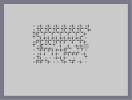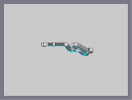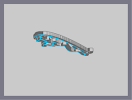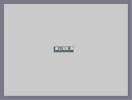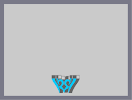Another, yet less frustrating, maze. Jag Test Jaguar Maserati Logo C Ya Dragon Test

## Comments

Pages: (0)

come back!

### holy shit...

that is impressive

### Wow

Bit small but wow

### TUT VID IS COMPLETE

I have finished my tutorial video.
I will make a time lapse of my work, but it will take time.
Here is the link:http://www.youtube.com/watch?v=6rY-5xS_1WU

lol

### And fire

That's not going to happen xD

### @karma

After this, he will submit 1 more map, then he's done.

That is all.

### Impressive.

I'd love to see more.

### could you possibly

make a map saying FBF XD

Faved.

### Wow

Never seen anything like this! Nice work!

### Yay!

You listened to me. :D. Glad to see you around. Nice work.

### camstudio

google it.
seriously, nice work, so is this a comeback or just submitting your archive?
say comeback :}

### There are...

exactly 361 thwumps and 2025 bounce blocks.
whoa, that's insane, nice work, how many thwumps are there?

Nice text.